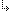- 2.2.12.6. -2. TMT Pascal Language Description2.2. Pascal Language Structure2.2.12. Procedures and Functions2.2.12.6. Using Statement as Procedure

## 2.2.12.6. Using Statement as Procedure

With TMT Pascal you can use any statement as a procedure body, except for the assignment and the procedure calls.

The RESULT variable in the body of such functions denotes the variable that contains the return value. The RESULT is of the function return type and may be used as a variable without any restrictions.

With TMT Pascal you can enter the procedure body directly as a procedure parameter. The procedure or function header (if not specified) takes the procedural parameter type. If the procedure header is specified, the procedure name is omitted.

Example:
```function Integral(function f(a: Real):Real;
low, high, step: Real): Real;
begin
...
end;
...
Writeln(integral (function(x: Real): Real;
begin Result := sqrt(x) end, 0, 10, 0.1));
Writeln(integral(begin Result := sqrt(a) end, 0, 10, 0.1));
Writeln(integral(function;     // function keyword needed
var x: Real;  // for local declaration
begin x := sqrt(a); Result := x end, 0, 10, 0.1)
);
Writeln(integral(declare;      // other way
var x: Real;  // for local variable declaration
begin
x := sqrt(a);
Result := x
end, 0, 10, 0.1)
);```
TMT Pascal allows exit from a local procedure to the one that contains it. This feature is listed in the Pascal's ANSI standard but not realized in Borland Pascal. Together with procedural values, this is very useful for error handling:
```program Test;
var
on_eof: procedure;
var c: char;

begin
if Eof (Input) then on_eof;
end;
procedure MyProc;
label eof_reached;
procedure go_eof;
begin
goto eof_reached;
end;
begin
on_eof := go_eof;

Eof_reached:
writeln ('*** EOF ***');
on_eof := nil;
end;
begin
MyProc;
end.```
Restriction: Break and Continue operators cannot be used for exit from a procedure. Use Goto instead.

Example:
```{ incorrect example }
for i := 1 to 10 do
Writeln ( integral(            // from previous example
if a < 0 then break  // incorrect
else result := sqrt (a), i, i + 1, 0.01)
);

{ correct example }
declare
label L;
begin
for i := 1 to 10 do Writeln (
integral(
if a < 0
then goto L  // correct
else result := sqrt (a), i, i + 1, 0.01)
);```
Functions may return any values of any type, including structures and arrays.

- 2.2.12.6. -